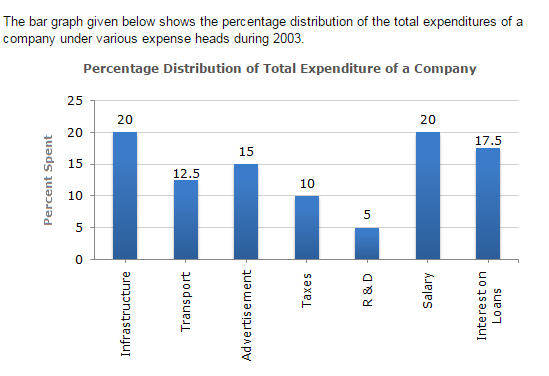Direction1.

The total amount of expenditures of the company is how many times of expenditure on research and development?

 A. 27 B. 20 C. 18 D. 8
Explanation: Let the total expenditures be Rs. x. Then, the expenditure on Research and Development (R & D) = Rs. (5% of x) = Rs. ( 5 x x ) 100 = Rs. ( x ) . 20 Therefore Ratio of the total expenditure to the expenditure on R & D = ( x ) x/20 = ( 20 ) . 1 Then, the total expenditure is 20 times the expenditure of Research and Development.

2.

If the expenditure on advertisement is 2.10 crores then the difference between the expenditure on transport and taxes is?

 A. Rs. 1.25 crores B. Rs. 95 lakhs C. Rs. 65 lakhs D. Rs. 35 lakhs
Explanation: Let the total expenditure be Rs. x crores. Then, 15% of x = 2.10 => x = ( 2.10 x 100 ) = 14. 15 Therefore Total expenditure = Rs. 14 crores and so, the difference between the expenditures on transport and taxes = Rs. [(12.5 - 10)% of 14] crores = Rs. [2.5% of 14] crores = Rs. 0.35 crores = Rs. 35 lakhs

3.

What is the ratio of the total expenditure on infrastructure and transport to the total expenditure on taxes and interest on loans?

 A. 5:4 B. 8:7 C. 9:7 D. 13:11
Explanation: Let the total amount of expenditures be Rs. x. Then, the total expenditure on infrastruture and transport = Rs. [(20 + 12.5)% of x] = Rs. [32.5% of x] = Rs. ( 32.5x ) 100 and total expenditure on taxes and interest on loans = Rs. [(10 + 17.5)% of x] = Rs. [27.5% of x] = Rs. ( 27.5x ) 100 Therefore Required ratio = ( 32.5x/100 ) = 13 . 27.5x/100 11

4.

If the interest on loans amounted to Rs. 2.45 crores then the total amount of expenditure on advertisement, taxes and research and development is?

 A. Rs. 7 crores B. Rs. 5.4 crores C. Rs. 4.2 crores D. Rs. 3 crores